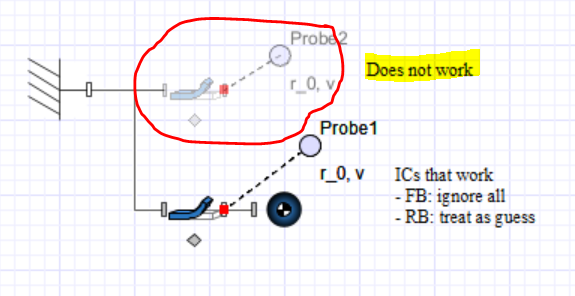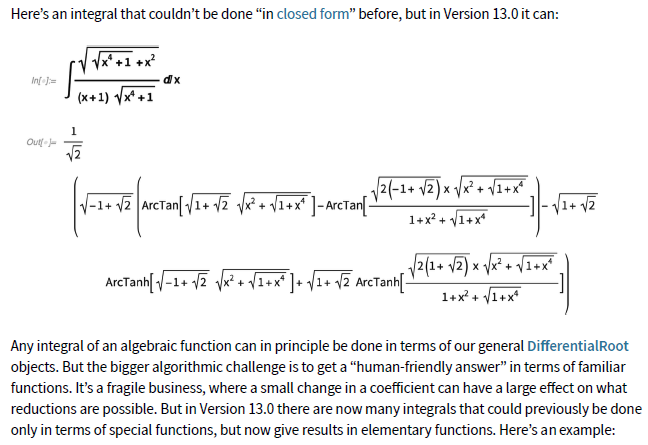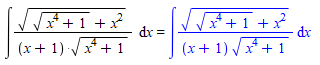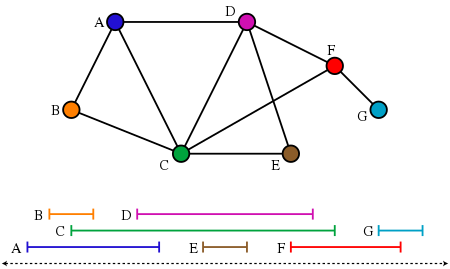## How to apply initial conditions to a flexible beam...

I was not able to apply ICs (initial conditions) using the advanced parameter settings of the flexible beam component in the attached file. My intention is to have a beam moving at t=0s (velocity of end frame <> 0). The computed initial values for the elastic coordinates (see output console for the disabled component below encircled in red) indicate that the flexible beam has no kinetic energy at t=0s.

A workaround is to attach a rigid body to the end frame and apply the ICs to the body. (Inertia and mass of the rigid body can be set to zero.)How to apply initial conditions to the disabled component so that it moves as the workaround?

Imposing_IC_v.msim

## Development work for closed form symbolic integrat...

At the end of 2021, Mathematica added capability for integration:

https://blog.wolfram.com/2021/12/13/new-in-13-symbolic-numeric-computation/Trying this in Maple, it does not look like it can solve it:My questions are then, is there a way to get Maple to solve this? Does Maplesoft actively work on solving more integral types and is it likely support for this integral will be added at some point?

## Find all possible subgraph isomorphic to H in a Gr...

Given Graph G and Graph H how to store the list of all the various Subgraphs of Shape H in G with their vertex labels.

I have write a code but it may not be the optimal way to do it and it may be time consuming for even medium graphs too

Any way to optimize

Let From this list of subgraphs H of say [G0,G1,G2,.....] we pick NumberOfVertices(G) Graphs
say H= [H0,H1,H2,H3,....,Hn]

i) Now any two graph Hi,Hj have excatly one edge interection if (i,j) is an edge in G

ii) An edge in  the list H will occur exactly twice only in the entire list of graphs

If these two conditions satifies all such H I need to save

Toycode until picking graphs

From that how to pick lists which satify conditions i) and ii) is another need help

Toy_code.mw

## How to solve this equation?...

How to solve this RLC Electric Circuit  with this initial conditions,I couldn't plot this equation.Help me to do this problem.

EC-2.mw

## Clipped view in plots...

Is there any setting that controls the extent of a plot?

Left hand plot has defined extent of the plot, while the plot on the right hand side has not. When panning the graphics on the right side the plot is clipped.

Any idea how to make Maple to use the whole extent of the plot component as a boundary?

## Is there a way to try to write this complicated fu...

I have this tedious looking function that I want to write in terms of the other expression but the command i usually use does not work here because the expressions are not polynomials. I am wondering if there is an alternative to doing this manually.
Temp.mw

## Using Forget in a loop?...

I would like to use the forget command to make maple forget all the things it remembers in each iteration of the loop.

Could someone help me with that?

More details:  I use ansatz to try to solve systems of pdes. sometimes i put the ansatzs in a list and run a loop to try to solve the set of pdes for each ansatz. Sometimes this takes up a lot of memory and maple says kernel connection is lost.

## How to solve large sparse systems of linear equati...

I have some large systems of linear equations.  The solutions are probability generating functions.  I can get solutions in a few minutes for systems of up to n= 200 eqns or so, but Maple just cycles indefinitely if I try to solve much larger systems.  I really only need to perform Gaussian Elimination, as I only need to solve for one of the n solutions.  The matrices are sparse, there are only 3 non-zero entries per row.  I tried to get help from the manuals but I get the impression that sparse solutions are only available for numeric computations.   Doesn't Maple allow for sparse symbolic solutions?  If so, how to do it?

## Error, (in dsolve/numeric/bvp) initial Newton iter...

Hi everyone
how can i overcome this error to solve this ODE ? tnx in advanced.

 > restart
 > U := 1:L := 10:k := 1:Dea := 0.00001:CA0 := 10:Pe := U*L/Dea:Da := k*CA0^2/Dea:
 > Eq1 := diff(CA(x), x, x) - Pe*diff(CA(x), x)/L = Da*L*CA(x)^2/CA0;(1)
 > BCs := CA(0) = CA0, D(CA)(L) = 0(2)
 > ans := dsolve([Eq1, BCs], numeric);
 >

## ShowSolution does not compute the indeterminate li...

In the following I show two functions f and g where ShowSolution(Limit(..)) works for f but not for g. Any idea why?

with(Student[Calculus1]);

f := x^2 + x:
limit(f, x = -infinity);
infinity
ShowSolution(Limit(f, x = -infinity), maxsteps = 100);
// step-by-step solution is produced

g := x^2 + 3*x:
limit(g, x = -infinity);
infinity
ShowSolution(Limit(g, x = -infinity), maxsteps = 100);
Error, (in Student:-Calculus1:-ShowSolution) unable to compute solution steps

Maple is able to change f into an equivalent expression by factoring out x^2, but does not seem to be able to do it with g.

I attach two screenshots that show this curious behaviour.

## The function IsIntervalGraph needs an additional ...

An interval graph is an undirected graph formed from a set of intervals on the real line, with a vertex for each interval and an edge between vertices whose intervals intersect. Recognizing interval graphs  is in linear time.Seven intervals on the real line and the corresponding seven-vertex interval graph.

`IsIntervalGraph(G)` (was introduced in Maple 2022) tests whether the graph G could be expressed as an interval graph for some collection of intervals. If a graph is an interval graph, then the intervals corresponding to its vertices should be given. However,  `IsIntervalGraph`does not provide such an option, which makes it impossible for me to verify the correctness of the results or see more information.

```with(GraphTheory):
G:=Graph({{1,2},{1,3},{1,4}, {4,2},{4,3}});
IsIntervalGraph(G)
```

true

Therefore, an option like the "certificate" option in SageMath needs to be provided.

```g = Graph({1: [2, 3, 4], 4: [2, 3]})
g.show()
g.is_interval()
g.is_interval(certificate=True)```(True, {1: (0, 5), 2: (4, 6), 3: (1, 3), 4: (2, 7)})

I have looked at the source code of `IsIntervalGraph`and it seems to be checking whether the complement graph is comparability. I am not sure if this transformation can still find the corresponding intervals.

```print(IsIntervalGraph)
proc(G::GRAPHLN)::truefalse;
local G2;
G2 := GraphTheory:-GraphComplement(G);
return GraphTheory:-IsComparabilityGraph(G2);
end proc

print(IsComparabilityGraph)
proc (G::GRAPHLN, { transitiveorientation::truefalse := false,

usecached::truefalseFAIL := FAIL }, ` \$`)::truefalse; local

iscomparability, L, A, result, V; A := op(4, G); result :=

FindTransitiveOrientation(A, transitiveorientation); if

result = NULL then false elif transitiveorientation then V

:= op(3, G); true, GraphTheory:-Graph(V, result) else true

end if end proc
```

By the way, can the  "FindTransitiveOrientation "  in the function `IsComparabilityGraph` be used by the user?

## Is it possible to upgrade some external library to...

https://www.maplesoft.com/support/help/Maple/view.aspx?path=copyright lists some external packages used by Maple, but it appears that certain libraries are of outdated (albeit not obsolete) versions. For example, Maple 2023 uses FLINT 2.6.3 (released in 2020), but the newest stable version of FLINT is 2.9.0. Also, Maple 2023 uses Z3 4.5.0 (released in 2016), but the newest stable version of Z3 is 4.12.1. In addition, Maple 2023 uses GCC 10.2.0 (released in 2020), but the newest stable version of GCC is 13.1. Since they are distributed under free licenses, I can download the most recent (or even nightly) release's source code, but how can I replace the old components that Maple uses by the latest ones by myself?

## Impliment of Artificial Neural Network ...

Hello,

Can we impliment Artificial Neural Network for nonlinear coupled ODE equation with boundary conditions.? In maple

I wont seen any post regarding ANN in mapleprime.

## How to access menus when window is maximized?...

There appears to be a bug in Maple 2023.0 (Build ID 1689885)  that does not allow you to access submenus when the window is maximized.  For example, trying to select "Insert --> Execution Group" does not work, nor does any other submenu (the submenu will not display and is not accessible).  The only workaround is to un-maximize the window, select the submenu (for example "Insert --> Execution Group --> Before Cursor"), and then re-maximize the window.  Everything works as it should as long as the window is not maximized.  This is on Microsoft Windows 11 with a 2160p monitor.  Any suggestions on how to fix this are appreciated.

## Solving sets of PDE perturbatively?...

I dont know if this is a well formulated question but if I had a set of PDES that have a constant appearing in them (say alpha), would there be a way to solve for the PDE such that the right had side is not zero but just has higher order order terms (say alpha^3 and higher).

For example consider (the random set of equations)

eq1 := alpha*diff(f(t, r), r,r) + diff(g(t, r), t) + alpha*g(t, r) = 0;

eq2 := alpha*diff(g(t, r), r) + diff(f(t, r), t,t) + f(t, r) = 0;

Is there a way to solve for the system of pde such that the right hand side is only terms of order alpha^3 and higher?

﻿